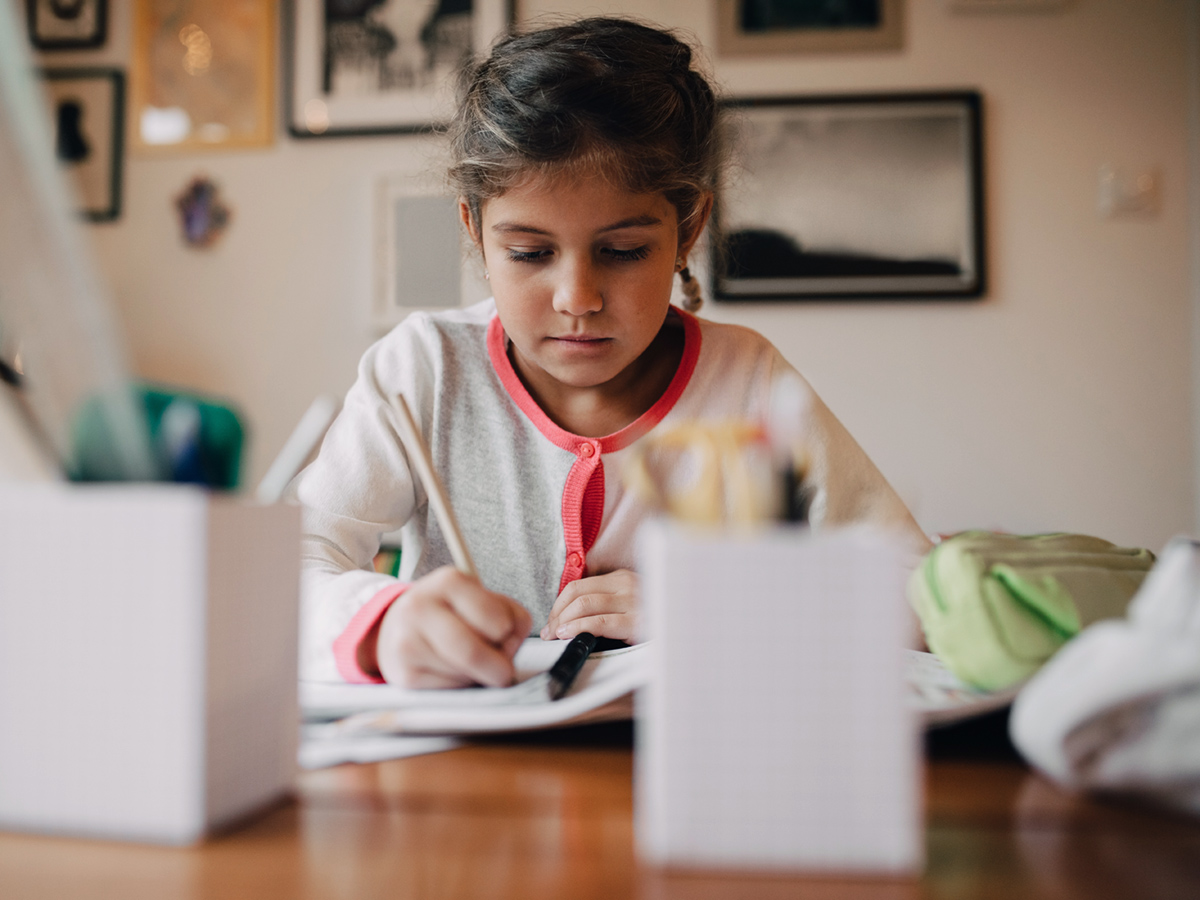# When do kids learn addition and subtraction?## ByTara Drinks## At a glance

• Learning to add and subtract doesn’t happen all at once.

• Kids need certain basic skills to do addition and subtraction.

• Some kids take longer to develop these skills than others.

Addition and subtraction are the first math operations kids learn. But it doesn’t happen all at once. Learning to add and subtract typically happens in small steps between kindergarten and the fourth grade.

## When do kids learn to add and subtract?

Learning to add and subtract starts with learning to count. For some kids, learning how to count begins in daycare or preschool. But this doesn’t mean kids know how to add or subtract by then. Here’s what learning to add and subtract typically looks like for kids:

In kindergarten: Kids count forward and backward, starting and ending with zero. Kids also start learning to join single digits and to compare amounts to see the difference.

In first grade: Kids join single-digit and double-digit numbers for addition. They also subtract single-digit numbers and 10s.

In second grade: Kids work on more complicated addition and subtraction. They also start learning regrouping, or “borrowing.”

By fourth grade: Kids typically understand how to add and subtract multi-digit numbers without using objects and pictures to model it. They can do regrouping, too.

When kids get to fourth grade and aren’t yet able to add and subtract without using objects and pictures, it doesn’t necessarily mean there’s a problem. Kids develop at different rates. But if they’re struggling and behind, it’s important to find out why.

## Why some kids struggle with addition and subtraction

Kids can have a hard time learning math for different reasons. A common one is trouble with a set of skills that are more basic than counting or addition and subtraction. These skills are known as number sense.

## Ways to help kids learn addition and subtraction

Practicing adding and subtracting can help to improve these skills. But it doesn’t have to feel like work. Here are some fun activities to try:

Visualize it. Use Cheerios or dried beans for counting. Moving them around is a great way for kids to understand how math operations work.

Make it art. Have kids use crayons or markers to show what the math operation looks like.

Play math board games. Games like Connect Four use concepts like counting and sequencing. Here are more board game ideas.

## Key takeaways

• By the fourth grade, most kids understand how to add and subtract.

• Playing math board games can help kids get better at adding and subtracting.

• If kids are behind and struggling at this point, it’s important to find out why.

## Share